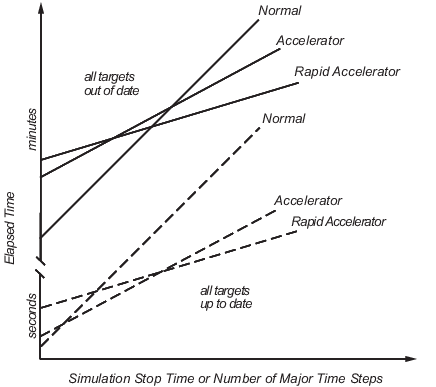## Comparing Performance

### Performance of the Simulation Modes

The Accelerator and Rapid Accelerator modes give the best speed improvement compared to Normal mode when simulation execution time exceeds the time required for code generation. For this reason, the Accelerator and Rapid Accelerator modes generally perform better than Normal mode when simulation execution times are several minutes or more. However, models with a significant number of Stateflow® or MATLAB Function blocks might show only a small speed improvement over Normal mode because in Normal mode these blocks also simulate through code generation.

Including tunable parameters in your model can also increase the simulation time.

The figure shows in general terms the performance of a hypothetical model simulated in Normal, Accelerator, and Rapid Accelerator modes.#### Performance When the Target Must Be Rebuilt

The solid lines in the figure show performance when the target code must be rebuilt (“all targets out of date”). For this hypothetical model, the time scale is on the order of minutes, but it could be longer for more complex models.

As generalized in the figure, the time required to compile the model in Normal mode is less than the time required to build either the Accelerator target or Rapid Accelerator executable. It is evident from the figure that for small simulation stop times Normal mode results in quicker overall simulation times than either Accelerator mode or Rapid Accelerator mode.

The crossover point where Accelerator mode or Rapid Accelerator mode result in faster execution times depends on the complexity and content of your model. For instance, those models running in Accelerator mode containing large numbers of blocks using interpreted code (see Select Blocks for Accelerator Mode) might not run much faster than they would in Normal mode unless the simulation stop time is very large. Similarly, models with a large number of Stateflow Chart blocks or MATLAB Function blocks might not show much speed improvement over Normal mode unless the simulation stop times are long.

For illustration purposes, the graphic represents a model with a large number of Stateflow Chart blocks or MATLAB Function blocks. The curve labeled “Normal” would have much smaller initial elapsed time than shown if the model did not contain these blocks.

#### Performance When the Targets Are Up to Date

As shown by the broken lines in the figure (“all targets up to date”) the time for the Simulink® software to determine if the Accelerator target or the Rapid Accelerator executable are up to date is significantly less than the time required to generate code (“all targets out of date”). You can take advantage of this characteristic when you wish to test various design tradeoffs.

For instance, you can generate the Accelerator mode target code once and use it to simulate your model with a series of gain settings. This is an especially efficient way to use the Accelerator or Rapid Accelerator modes because this type of change does not result in the target code being regenerated. This means the target code is generated the first time the model runs, but on subsequent runs the Simulink code spends only the time necessary to verify that the target is up to date. This process is much faster than generating code, so subsequent runs can be significantly faster than the initial run.

Because checking the targets is quicker than code generation, the crossover point is smaller when the target is up to date than when code must be generated. This means subsequent runs of your model might simulate faster in Accelerator or Rapid Accelerator mode when compared to Normal mode, even for short stop times.

### Measure Performance

You can use the `tic`, `toc`, and `sim` commands to compare Accelerator mode or Rapid Accelerator mode execution times to Normal mode.

2. On the Simulation tab, in the Simulate section, select `Normal` from the drop-down menu.

3. Use the `tic`, `toc`, and `sim` commands at the command line prompt to measure how long the model takes to simulate in Normal mode:

`tic,[t,x,y]=sim('myModel',10000);toc`

`tic` and `toc` work together to record and return the elapsed time and display a message such as the following:

`Elapsed time is 17.789364 seconds.`

4. On the Simulation tab, in the Simulate section, select `Accelerator` or `Rapid Accelerator` from the drop-down menu. Build an executable for the model by clicking Run. The acceleration modes use this executable in subsequent simulations as long as the model remains structurally unchanged. Code Regeneration in Accelerated Models discusses the things that cause your model to rebuild.

5. Rerun the compiled model at the command prompt:

`tic,[t,x,y]=sim('myModel',10000);toc`

6. The elapsed time displayed shows the run time for the accelerated model. For example:

```Elapsed time is 12.419914 seconds. ```

The difference in elapsed times (5.369450 seconds in this example) shows the improvement obtained by accelerating your model.